# Dividing Fractions

Division indicates how many times one quantity is contained in another quantity. For example, in the illustration below, you can see that the whole number 2 contains 6 thirds.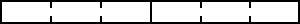Since 2 contains 6 one-thirds, we can say that 2 divided by one-third is 6.

 so 2 ÷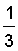= 6 and 2 x 3 = 6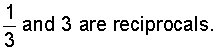As you can see, dividing a first fraction by a second, nonzero fraction is the same as multiplying the first fraction by the reciprocal of the second fraction. This leads us to the following procedure.

Procedure: To divide a first fraction by a second, nonzero fraction, multiply the first traction by the reciprocal of the second fraction.

This method of dividing fractions is also referred to as invert and multiply since we are inverting the divisor and then multiplying. Basically, we are changing the division problem to a multiplication problem after inverting the divisor. This allows us to multiply the first fraction by the reciprocal of the second fraction.

Example 1: Divide.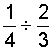Analysis: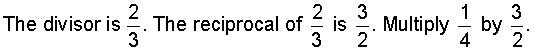Solution: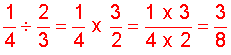Example 2: Divide.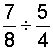Analysis: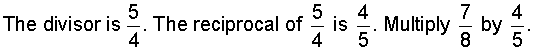Solution:In example 2, it was necessary to divide out common factors.

Example 3: Divide.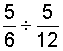Analysis: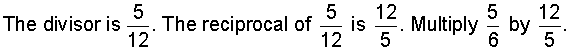Solution:In example 3, it was necessary to simplify the result.

Example 4: Divide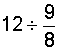Analysis:Solution: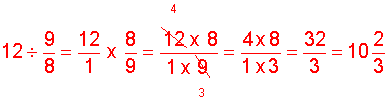Example 5: A candy bar is 3/4 of an inch long. If it is divided into pieces that are 1/8 of an inch long, then how many pieces is that?

Analysis: To solve this problem, we will divide 3/4 by 1/8.

Solution:Answer: There will be 6 pieces of candy.

Summary: To divide a first fraction by a second, nonzero fraction, multiply the first fraction by the reciprocal of the second fraction. Simplify the result, if necessary.

### Exercises

Directions: Divide the fractions in each exercise below. Be sure to simplify your result, if necessary. Click once in an ANSWER BOX and type in your answer; then click ENTER. After you click ENTER, a message will appear in the RESULTS BOX to indicate whether your answer is correct or incorrect. To start over, click CLEAR.

Note: To write the fraction three-fourths, enter 3/4 into the form. To write the mixed number four and two-thirds, enter 4, a space, and then 2/3 into the form.

 1.ANSWER BOX:   RESULTS BOX:
 2.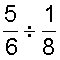ANSWER BOX:   RESULTS BOX:
 3.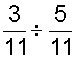ANSWER BOX:   RESULTS BOX:
 4.ANSWER BOX:   RESULTS BOX:
 5. Justin gave 2/3 of his pizza to 4 friends who shared the pizza equally. What fraction of the original pizza did each friend get? ANSWER BOX:     RESULTS BOX:

 Lessons on Multiplying and Dividing Fractions and Mixed Numbers 1. Multiplying Fractions 2. Multiplying Fractions by Cancelling Common Factors 3. Multiplying Mixed Numbers 4. Reciprocals 5. Dividing Fractions 6. Dividing Mixed Numbers 6. Solving Word Problems 7. Practice Exercises 8. Challenge Exercises 9. Solutions